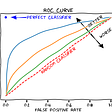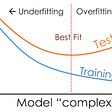# Testing for significance of Regressors

F test

This test is used for checking if all the coefficients of the regression are collectively equal to 0 or not.

For this test we have defined two models

1. Restricted model — In this model, the coefficients of all the explanatory variables are 0
2. Unrestricted model — In this model, the coefficients of all the explanatory variables are not 0

Equation of restricted model

Equation of unrestricted model

Now we calculate R squared of both restricted and unrestricted model

Then we calculate sum of squared errors for both restricted and unrestricted models

Where e and u are the errors of unrestricted and restricted models respectively

Then we define the F statistic as follows

Which can be written as

The F statistic follows a F distribution with n-1 degrees of freedom

Hypothesis of F test

If the F test is accepted, i.e. the p-value of the F statistic is greater than 0.05, it signifies that all the coefficients of your regressors are 0 and you should look for a different set of explanatory variable or try fitting a non linear model

T test

This test checks if the coefficient of an individual regressor is 0 or not

Here we define our model as

Now for an individual coefficient, we define the t statistic as

The hypothesis of T test is

If the null hypothesis of T test is accepted, it means that the coefficient is 0 and it does not contribute much to the model so it should be either removed from the model or replaced with some other explanatory variable

# Testing for linearity in the data set

For checking linearity, we can do the following

1. Check for linearity of features by a scatter plot of each feature if possible
2. Perform Ramsay Reset test to check for linearity of the dataset

Ramsay reset test

This test checks for the polynomial dependence of the target variable on the explanatory variables

We can regress the model as

As we know y hat encloses all the possible combinations of beta and raising y hat to a power will take care of all the expansion terms, we can re write the equation of y hat as

Now we check if the coefficients of all the powers of y hat greater than 2 are 0 or not. We check from the power of 2 because the power of 1 is excused as we have allowed linear relationship in the model. For checking the coefficients, we use F test

Hypothesis of Ramsay Reset test

If the null hypothesis is accepted, it signifies that the data is linear

# Assumptions of Gauss Markov Setup

As we may remember, the assumptions of Gauss Markov setup were that

1. The regressors are independent
2. The residuals belong to a normal distribution
3. The residuals are homoscedastic

To test these assumptions, we have the following tests

# Validating the assumption of normality of residuals

To validate the assumption of normality we have the following tests.

Jarque Bera test

This test makes use of the fact that the skewness of a normal distribution is 0 and the kurtosis of a normal distribution is 3. To test these two characteristics simultaneously, we have designed a Jarque Bera test statistic which can be given as follows

Points to remember

1. The constants are divided so that the JB score fits the chi squared distribution
2. If skewness = 0 and kurtosis = 3 then JB = 0 else JB is not equal to 0

Hypothesis is JB test

If the null hypothesis is accepted, it signifies that the residuals follow a normal distribution

Quantile Quantile plot

This test makes use of the fact that the quantiles of our residuals should be equal to the quantiles of a normal distribution if our residuals are following a normal distribution

If the quantiles are equal, then the sample quantiles (quantiles of our residuals) plotted against the theoretical quantiles (quantiles of a normal distribution) should lie along the line y=x (i.e with a slope of 45 degree)

Kolmogoro Smirnov Test

This test makes use of the fact that the cumulative distribution function of a normal distribution matches the cumulative distribution function of our residuals, hence signifying that the supremum of the difference between the cumulative distribution function of a normal distribution and the cumulative distribution function of our residuals should be equal to 0

The hypothesis of KS test

If the null hypothesis is accepted, it signifies that the residuals follow a normal distribution

Cramer Von Misses Statistic

This test makes use of the fact that the integration of the squared difference between the cumulative distribution function of a normal distribution and the cumulative distribution function of our residuals should be 0

Hypothesis of CV test

If the null hypothesis is accepted, it signifies that the residuals follow a normal distribution

Anderson Darling Test

This test makes use of the fact that the weighted integration of the squared difference between the cumulative distribution function of a normal distribution and the cumulative distribution function of our residuals should be 0

If the null hypothesis is accepted, it signifies that the residuals follow a normal distribution

# Validating Assumption of Homoscedasticity of residuals

Breusch Pagan test

For a model

We can regress the variance as

If the variance is homoscedastic, it should depend on any form of X. To verify that we conduct F test on all the coefficients from delta 1 onwards

Hypothesis of BP test

If the null hypothesis is accepted, it signifies that the residuals follow a normal distribution

Breusch Pagan test only tests for linear relationships between the regressors and the variance so it fails to detect polynomial relationships between the variance and regressors (if any) hence to overcome that we use White test

White Test

This test checks for linear as well as polynomial relationship between the variance and regressors

For a model

We can regress the variance as

As we know y hat encloses all the possible combinations of beta and raising y hat to a power will take care of all the expansion terms, we can re write the equation of variance as

The hypothesis of White test are:

If the null hypothesis is accepted, it signifies that the residuals follow a normal distribution. Here we are testing for non linearity up to the power of p where p is a real number

# General inference for the tests

1. The tests relating to significance of regressors should get rejected as it will signify that the regressors that we have chosen are significant in predicting the target variable
2. The tests relating to the linearity of dataset should get accepted as it will signify that the data is linear and we can proceed with applying linear regression algorithm to the dataset
3. The tests relating to normality of residuals should get accepted as it will signify that the residuals are normally distributed which satisfies the assumptions of the Gauss Markov setup under which the linear regression model was built
4. The tests related to homoscedasticity of residuals should get accepted as it will signify that the residuals are homoscedastic which satisfies the assumptions of the Gauss Markov setup under which the linear regression model was built

--

--

--

A data science enthusiast currently doing bachelor's degree in data science

Love podcasts or audiobooks? Learn on the go with our new app.

## Amazon Fine Food Reviews## Using AI To Compare the Effectiveness of Lockdown Procedures## Ace the Data Science Interview## A ClearQuote Case Study : Popular Hyundai## How To Convert ‘datetime’ To Separate Year, Month, Day, Hours, Minutes, Seconds In Python## Analytics Dashboards Are Dead: This is the Future of A.I.## Analysis of the Iris dataset using Artificial Intelligence## Applying Machine Learning To The E.Coli Class Imbalance Dataset## Aayushmaan Jain

A data science enthusiast currently doing bachelor's degree in data science

## A Quick Glance at Machine Learning in Data Science:## Song Popularity Prediction## Exploration of Titanic Survival Using Decision Trees## Basics understanding of Regression in Machine Learning